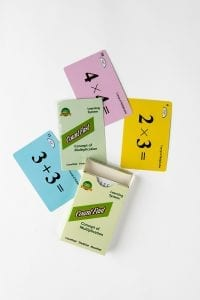# Concept of +- (1-6) Lesson Plan

####OVERVIEW & PURPOSE

Week 2 of the 1st Grade CountFast program focuses on addition and subtraction using the digits 0 through 6.  This module includes lessons on how to play an addition and subtraction game using the card deck.  Spend 15 minutes each day on one of the activities listed in this module.  Card decks should go home with students each day for additional practice with a parent at home. Each week, a new deck is introduced and the previous deck is for the student to keep at home for continued practice.

#### EDUCATION STANDARDS

1. NCTM Standard: develop and use strategies for whole-number computations, with a focus on addition and subtraction
2. NCTM Standard: understand the effects of adding and subtracting whole numbers
3. MATH.CONTENT.K.OA.A.1:  Represent addition and subtraction with objects, fingers, mental images, drawings1, sounds (e.g., claps), acting out situations, verbal explanations, expressions, or equations.
4. MATH.CONTENT.1.OA.D.7:  Understand the meaning of the equal sign, and determine if equations involving addition and subtraction are true or false.

#### OBJECTIVES

1. Sort the numbers zero through twelve in least-to-greatest and greatest-to-least form.
2. Create accurate addition and subtraction equations using the numbers 0-6.
3. Understand and use the symbols for equal, add (plus), and subtract (minus).

#### MATERIALS NEEDED

1. One CountFast Concept of +/- (1-6) card deck for each student.  This deck is for school and home use.  Discuss routine and expectations for taking home the deck and returning it to school each day.
2. One writing utensil per student

#### Concept of +/- (1-6) – Day 1

Teacher Model/Direct:  Use the yellow cards from the deck.  These have the numbers 0-12 written as digits and as words.  You will also find a card for addition (plus), subtraction (minus), and equal.  Review sorting the cards from least to greatest, and greatest to least.  Explain to students that tomorrow they will be playing an addition game using the yellow cards.  This is also a great time to review with “How many more?” and “How many fewer?” questions, using the cards as a number line.

Student Activity:  Give each student a deck and ask them to take out the yellow cards.  Have students lay out the numbers 0-12 in order in front of them.  Touching each card one at a time, say the numbers in order from least to greatest.  Repeat procedure but say the numbers in order from greatest to least.  Work with a partner to ask/answer “how many more” and “how many fewer” questions.

Home Activity: Students will take home the deck and the “CountFast Home Connection” letter.  Students will review the yellow cards with parent(s).  Parents will work with child to put the numbers in order from least to greatest, and from greatest to least.

#### Concept of +/- (1-6) – Day 2 AND 3

Teacher Model/Direct:  Introduce the addition equation game.  Ask students to observe their blue cards and notice that there are two sets of the numbers 0-6, as well as an addition and an equal sign. Call out addition equations for students to build with their cards (Ex:  2 + 3 =).  Have students complete the equation with a card showing the correct answer (5).  Talk about how to prove that the equation is correct.  While counting up, talk about the Commutative Property of Addition and how that property can be used to make counting up easier. (Ex:  it might be easier for the student to do  3 + 2 instead of 2 + 3).  Practice building and solving equations using the numbers 0-6.

Student Activity:  Have students work with a partner.  Using one set of blue cards, partners should take turns building accurate equations of their choice (or the partner can call out an equation to solve).  Partners should check each other’s accuracy and have a strategy for proving results.  Play until each partner has completed 7 different equations.  Each time the game is played, students should be building/solving equations faster because repetition builds memory.

Home Activity: Students will play the addition equation game with parents and use the ‘Addition Time Record’ card to keep track of how long it takes to create 7 accurate equations.

Concept of +/- (1-6) – Day 4 AND 5

Teacher Model/Direct: Introduce the pink cards in the deck.  Review the concept of subtraction as a smaller amount being taken away from a larger amount.  The pink cards have the minuends (larger #’s) of 7-12, as well as the numbers 0-6 which can be taken away from those minuends.  Play the subtraction equation game.  Call out subtraction equations (ex: 11 – 4 =) for students to build using the pink cards.  Check to see that students are able to put the correct answer (7).  Discuss whether or not Commutative Property can be applied to subtraction. (Can we do 4 – 11?  Why not?)

Student Activity:  Have students work with a partner.  Using one set of pink cards, partners should take turns building accurate subtraction equations of their choice (or the partner can call out an equation to solve).  Partners should check each other’s accuracy and have a strategy for proving results.  Play until each partner has completed 7 different equations.  Each time the game is played, students should be building/solving equations faster because repetition builds memory.

Home Activity: Students will play the subtraction equation game with parents and use the ‘Subtraction Time Record’ card to keep track of how long it takes to create 7 accurate equations.

#### Instructional Video

Stay SharpFree flash card decks to keep kids entertained and sharp!

To help you keep your kids math sharp during this crisis, we're giving you 3 free CountFast flash card decks for just \$6 in shipping.  Just have them take the quick quiz for their grade at the link below and you'll get a discount code to use on any three decks.